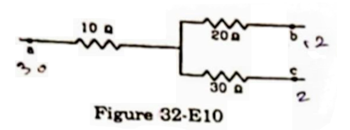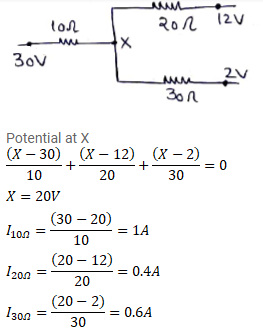# Figure (32-E10) shows a part of an electric circuit.Question:

Figure (32-E10) shows a part of an electric circuit. The potentials at the points $a$, $b$ and $c$ are $30 \mathrm{~V}, 12 \mathrm{~V}$ and $2 \mathrm{~V}$ respectively. Find the currents through the three resistors.Solution: